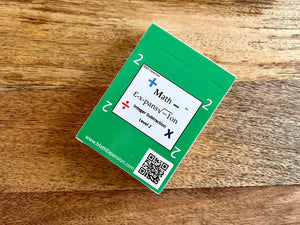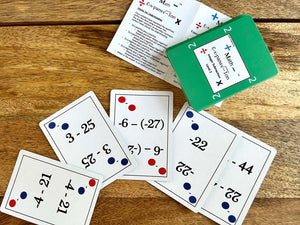# Integer Subtraction Level 2 Card Deck

Regular price \$19.00
Unit price  per

Looking for a fun and interactive way to help your scholars excel in math? Look no further! Math Expansion breaks down subtracting positive and negative numbers ("integers") using a hands-on minds-on method. This allows scholars to understand the concept of integers instead of memorizing rules. Scholars' skills are tested in Level 2 with two-digit numbers. Differentiate your classroom and have loads of fun during family game night while preparing scholars for algebra, college, and beyond!

Integer Subtraction Level 2 solidifies the scholars’ knowledge using 2-digit integers.

Each deck of Integer Subtraction Level 2 includes the following:

• A booklet explaining how to use integer counters and how to conduct checks for understanding
• A deck of 52 cards with more challenging integer subtraction problems and answers